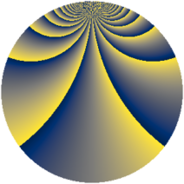# Properties

 Label 2736.2.hcLevel $2736$ Weight $2$ Character orbit 2736.hc Rep. character $\chi_{2736}(53,\cdot)$ Character field $\Q(\zeta_{36})$ Dimension $1920$ Sturm bound $960$

# Related objects

## Defining parameters

 Level: $$N$$ $$=$$ $$2736 = 2^{4} \cdot 3^{2} \cdot 19$$ Weight: $$k$$ $$=$$ $$2$$ Character orbit: $$[\chi]$$ $$=$$ 2736.hc (of order $$36$$ and degree $$12$$) Character conductor: $$\operatorname{cond}(\chi)$$ $$=$$ $$912$$ Character field: $$\Q(\zeta_{36})$$ Sturm bound: $$960$$

## Dimensions

The following table gives the dimensions of various subspaces of $$M_{2}(2736, [\chi])$$.

Total New Old
Modular forms 5856 1920 3936
Cusp forms 5664 1920 3744
Eisenstein series 192 0 192

## Trace form

 $$1920q + O(q^{10})$$ $$1920q - 24q^{10} + 24q^{16} + 960q^{49} + 96q^{52} + 144q^{70} + 96q^{76} + 192q^{85} + O(q^{100})$$

## Decomposition of $$S_{2}^{\mathrm{new}}(2736, [\chi])$$ into newform subspaces

The newforms in this space have not yet been added to the LMFDB.

## Decomposition of $$S_{2}^{\mathrm{old}}(2736, [\chi])$$ into lower level spaces

$$S_{2}^{\mathrm{old}}(2736, [\chi]) \cong$$ $$S_{2}^{\mathrm{new}}(912, [\chi])$$$$^{\oplus 2}$$